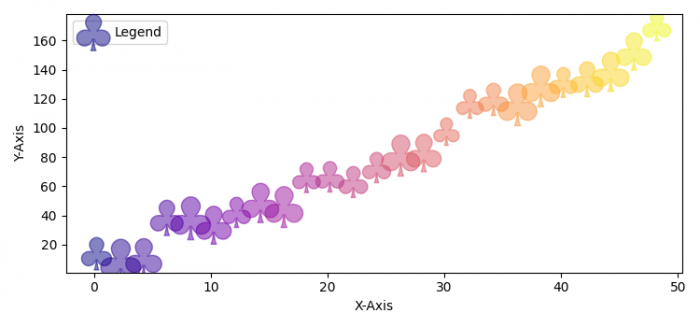# Plotting scatter points with clover symbols in Matplotlib

To plot scatter points with clover symbols in Matplotlib, we can take the following steps −

• Set the figure size and adjust the padding between and around the subplots.
• Create x, y and z data points using numpy.
• Plot x, y and s using scatter() method.
• Set X and Y axes labels.
• Place a legend at the upper left of the plot.
• To display the figure, use show() method.

## Example

import matplotlib.pyplot as plt
import numpy as np

plt.rcParams["figure.figsize"] = [7.50, 3.50]
plt.rcParams["figure.autolayout"] = True

x = np.arange(0.0, 50.0, 2.0)
y = x ** 1.3 + np.random.rand(*x.shape) * 30.0
s = np.random.rand(*x.shape) * 800 + 500

plt.scatter(x, y, s, c=x, alpha=0.5, marker=r'$\clubsuit$',
label="Legend", cmap="plasma")

plt.xlabel("X-Axis")
plt.ylabel("Y-Axis")

plt.legend(loc='upper left')

plt.show()

## Output